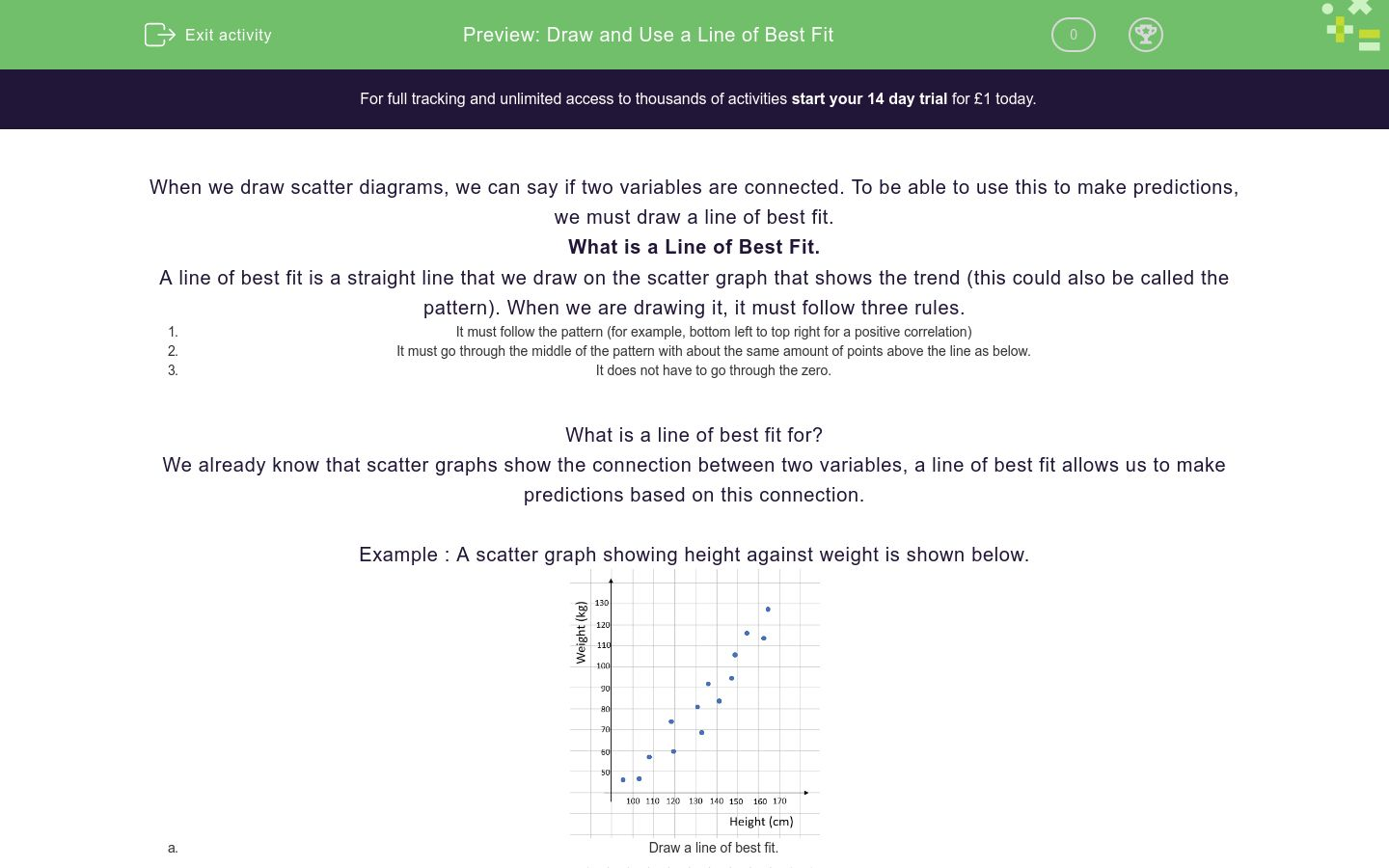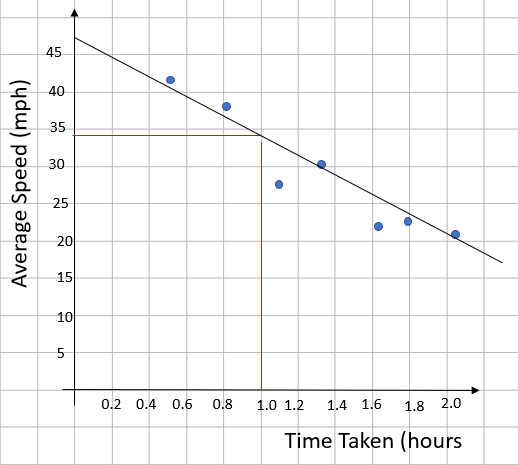# Draw and Use a Line of Best Fit

In this worksheet, students will learn to draw and use a line of best fit for a scatter diagram.Key stage:  KS 4

GCSE Subjects:   Maths

GCSE Boards:   AQA, Eduqas, Pearson Edexcel, OCR

Curriculum topic:   Statistics

Curriculum subtopic:   Statistics, Analysing Data

Difficulty level:### QUESTION 1 of 10

When we draw scatter diagrams, we can say if two variables are connected. To be able to use this to make predictions, we must draw a line of best fit.

What is a Line of Best Fit.

A line of best fit is a straight line that we draw on the scatter graph that shows the trend (this could also be called the pattern). When we are drawing it, it must follow three rules.

1. It must follow the pattern (for example, bottom left to top right for a positive correlation)
2. It must go through the middle of the pattern with about the same amount of points above the line as below.
3. It does not have to go through the zero.

What is a line of best fit for?

We already know that scatter graphs show the connection between two variables, a line of best fit allows us to make predictions based on this connection.

Example : A scatter graph showing height against weight is shown below.1. Draw a line of best fit.Following the rules we discussed earlier. The line of best fit goes through the middle of the pattern, has a similar amount of points above and below the line (7 above, 6 below and 1 on the line) and doesn’t go through the origin.

1. A man is 125 cm tall, predict how much he weighs.

We know the man is 125cm tall, so we draw a line up from 125 cm to the line of best fit, we then draw across the weight axis. We can predict that he weights about 75-76 kg1. A woman weighs 100 kg. How tall is she?

This is very similar to the previous question but this time we start from the weight axis, go across to the line of best fit and then read down to height. We can say she is about 147 cm tall.It’s absolutely vital to remember that as you are reading off a graph, you will always get a bit of a margin for your answers.

The trend line on a scatter graph shows the general pattern. This is called the...

The three rules for drawing a line of best fit are…

Which of the following graphs would be suitable to draw a LOBF on?A

B

C

D

Which of these lines of best fit have been drawn correctly?A

B

C

D

For the scatter graph shown, find the height of a woman who weighs 60 kg.113

120

For the scatter graph shown, find the weight of a man who is 1.5m tall.103 kg

105 kg

107 kg

The value of a type of car was tracked over time. This produced this scatter graph.

Estimate the value of a 4 year old car. Give your answer to the nearest £100 (Do not put units in your answer)Estimate the age of a can that is worth £6000. Give your answer to the nearest number of years.The time taken for a car journey and the average speed are plotted. By using a line of best fit, estimate the average speed for a 1 hour journey. Give your answer to the nearest whole number.I draw a scatter graph of Height against IQ (intelligence Quotient).

Will I be able to use this to predict the height of someone with an IQ of 120?

Yes

No

We dont have enough information

• Question 1

The trend line on a scatter graph shows the general pattern. This is called the...

EDDIE SAYS
When we are drawing a scatter graph, the points virtually never line up exactly. Because of this, we have to fit a line in as best as we can. This leads to the phrase line of best fit.
• Question 2

The three rules for drawing a line of best fit are…

EDDIE SAYS
These will become second nature for you. It’s vital you get this right as there is a mark for it in the exam. Remember that as you are drawing, there will be a range of answers that are acceptable.
• Question 3

Which of the following graphs would be suitable to draw a LOBF on?A
D
EDDIE SAYS
The reason we cannot draw a LOBF on B is that these lines have to follow the pattern of the points. As B has no correlation, it has no pattern to follow. Because C is a cumulative frequency, we cannot draw a line of best fit.
• Question 4

Which of these lines of best fit have been drawn correctly?A
D
EDDIE SAYS
To be drawn correctly, the lines of best fit have to follow the three rules. 1) It must follow the pattern (for example, bottom left to top right for a positive correlation) 2) It must go through the middle of the pattern with about the same amount of points above the line as below. 3) It does not have to go through the zero. Graph B violates rule 1 and graph C violates 2 and 3
• Question 5

For the scatter graph shown, find the height of a woman who weighs 60 kg.113
EDDIE SAYS
If we use our line of best fit.We can see that a 60 kg woman is about 113 cm tall
• Question 6

For the scatter graph shown, find the weight of a man who is 1.5m tall.105 kg
EDDIE SAYS
If we use our line of best fit.We can see that a man who is 150 cm tall should weigh about 105 kg
• Question 7

The value of a type of car was tracked over time. This produced this scatter graph.

Estimate the value of a 4 year old car. Give your answer to the nearest £100 (Do not put units in your answer)9200
9300
9400
9500
EDDIE SAYS
If we draw a line up from 4 years on our scatter graph, we get…Remember that you will get an allowed range. For this one you would get the marks if your answer was £9200 - £9500
• Question 8

Estimate the age of a can that is worth £6000. Give your answer to the nearest number of years.5
5.5
6
EDDIE SAYS
Drawing a line across from £6000 we get…This hits at about half way between 5 and 6 years so, because the question asks for the nearest year, you would get the mark for 5 years or 6 years.
• Question 9

The time taken for a car journey and the average speed are plotted. By using a line of best fit, estimate the average speed for a 1 hour journey. Give your answer to the nearest whole number.32
33
34
EDDIE SAYS
There are two steps for this one. Firstly we have to draw a line of best fit and then read off the speed for a 1 hour journey. Your scatter graph and working should look like this…This would give an answer in the range 32-34 mph
• Question 10

I draw a scatter graph of Height against IQ (intelligence Quotient).

Will I be able to use this to predict the height of someone with an IQ of 120?

No
EDDIE SAYS
You really have to think about this. Is how intelligent someone is linked to how tall they are? The scatter graph will have no correlation, so we won’t be able to draw a line of best fit.
---- OR ----

Sign up for a £1 trial so you can track and measure your child's progress on this activity.

### What is EdPlace?

We're your National Curriculum aligned online education content provider helping each child succeed in English, maths and science from year 1 to GCSE. With an EdPlace account you’ll be able to track and measure progress, helping each child achieve their best. We build confidence and attainment by personalising each child’s learning at a level that suits them.

Get started